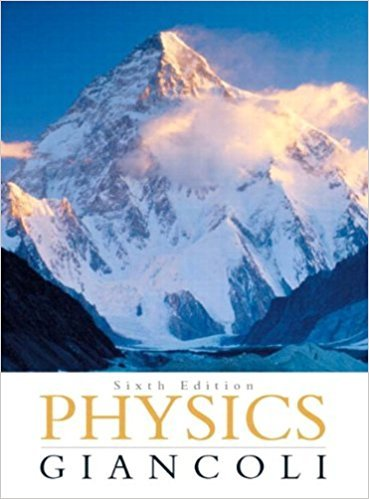×
×

# Answer: What is the magnitude of the acceleration of aISBN: 9780321569837 99

## Solution for problem 6 Chapter 5

Physics: Principles with Applications | 6th Edition

• Textbook Solutions
• 2901 Step-by-step solutions solved by professors and subject experts
• Get 24/7 help from StudySoup virtual teaching assistantsPhysics: Principles with Applications | 6th Edition

4 5 1 352 Reviews
11
1
Problem 6

What is the magnitude of the acceleration of a speck of clay on the edge of a potters wheel turning at 45rpm (revolutions per minute) if the wheels diameter is 32 cm?

Step-by-Step Solution:
Step 1 of 3

Class – 9/9/2016, Kinematics Dr. Ilia Gogoladze th Physics by Walker, 4 edition Instantaneous Velocity - V = lim →0 - SI unit: meter per second, m/s - This means that we evaluate the average velocity over a shorter and shorter periods of time; as that time becomes infinitesimally...

Step 2 of 3

Step 3 of 3

##### ISBN: 9780321569837

The answer to “What is the magnitude of the acceleration of a speck of clay on the edge of a potters wheel turning at 45rpm (revolutions per minute) if the wheels diameter is 32 cm?” is broken down into a number of easy to follow steps, and 32 words. Physics: Principles with Applications was written by and is associated to the ISBN: 9780321569837. This textbook survival guide was created for the textbook: Physics: Principles with Applications, edition: 6. The full step-by-step solution to problem: 6 from chapter: 5 was answered by , our top Physics solution expert on 09/09/17, 04:43AM. This full solution covers the following key subjects: acceleration, clay, diameter, edge, magnitude. This expansive textbook survival guide covers 33 chapters, and 4141 solutions. Since the solution to 6 from 5 chapter was answered, more than 304 students have viewed the full step-by-step answer.

Unlock Textbook Solution